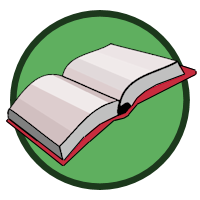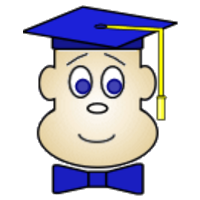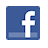Games
Problems
Go Pro!

# Four Digit Number

Pro Problems > Math > Number and Quantity > Number Theory > Digits

## Four Digit Number

I am a four digit number.

The sum of my digits is 20.

The product of my digits is 600.

The difference between my first two digits is 2, and the sum of my middle two digits is 11.

What number am I?

Presentation mode
Problem by Mr. Twitchell

## Solution

In order to make it feasible for teachers to use these problems in their classwork, no solutions are publicly visible, so students cannot simply look up the answers. If you would like to view the solutions to these problems, you must have a Virtual Classroom subscription.Assign this problem

## Similar Problems

### Three Digit Difference

Two positive integers, A and B, both have 3 digits. A is bigger than B. A – B is between 300 and 400. What is the value of A - B?

### Digits in a Multiplication Problem

You must use each of the integers from 0 to 5 exactly once to fill in the blanks in the multiplication problem below.

_ _ _ x _ _ x _ =

What is the largest possible value you can create?

### Set of Five Digit Numbers

S is the set of five-digit numbers such that the digits are in ascending order, there are no repeated digits, the sum of the first two digits is equal to the third digit, and the sum of the third and fourth digits is equal to the two more than the fifth digit. How many elements are in the set S? (Note that the leading digit cannot be a zero).

### Reverse Me

I'm a three digit number. Reverse my digits and subtract, and the result is 198. Reverse my digits and add, and the result is 1272.

What number am I?

### Three Digits, sum and product

I'm a three digit number. My first two digits multiply to 12, and my last two digits add to 14. What number am I?

### Back to Back

X is a three-digit number. Y is the number obtained when the digits of X are reversed. Z is the six-digit number obtained by writing X and Y back to back, with X written first. W is the six-digit number obtained by writing Y and X back to back, with Y written first. What is the largest number which the sum of Z and W must be divisible by?

### Sum of Digits

Find the sum of all the integers between one and 100 which have 14 as the sum of their digits.

### All My Digits

All my digits are non-zero perfect squares. If you treat my first two digits as a two-digit number, and treat my last two digits as a two-digit number, the sum of these two numbers is also a perfect square. If I am a three digit number, what numbers could I be?

Find the smallest positive integer which must be added to 30504 so that the resulting number is a palindrome.

Note: a palindrome is a number in which the digits would read the same forward and backward.

### Three Digit Number

I am thinking of a three-digit number. The sum of my digits is 17. Two of my digits add to 10, and two of my digits are the same. Find all possible values for my number.

Happy New Year, Rhonda's Zip Code, Three Digits with Difference, My Three Digits, Coffee Math, Three Digit Number, Two Digit Pattern Matching, Fill in the blanks, Grapes on the Vine, The Middle Palindrome, I Have Three Digits, Five Digit Number, Find the Number, Fiona's Telephone Number# Featured Resources on This Site

Create Christmas ornaments and more
Print fun mazes in many different shapes

# Blogs on This SiteReviews and book lists - books we love!The site administrator fields questions from visitors.Like us on Facebook to get updates about new resources